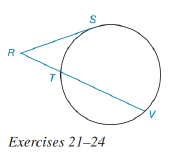Chapter 6.3, Problem 23E### Elementary Geometry for College St...

6th Edition
Daniel C. Alexander + 1 other
ISBN: 9781285195698

#### Solutions

Chapter
Section### Elementary Geometry for College St...

6th Edition
Daniel C. Alexander + 1 other
ISBN: 9781285195698
Textbook Problem
1 views

# In the figure for Exercises 21 to 24, R S ¯ is tangent to the circle at S. See Theorem 6.3.7.Given: R S ¯ ≅ T V ¯     a n d     R T = 6 Find: RS.(HINT: Use the Quadratic Formula.)To determine

To find:

To find RS.

Explanation

Given that, RS¯TV¯andRT=6.

The diagrammatic representation is given below,

Theorem:

If a tangent segment and a secant segment are drawn to a circle from an external point, then the square of the length of the tangent equals the product of the length of the secant with the length of its external segment.

By using the theorem 6.3.7 to get the following,

RS2=RVRT

We know that

RV=RT+TVRV=6+TVRV=6+RS. Since RS¯TV¯

Substitute the values RT=6,RV=6+RS in the above equation to get the following,

RS2=(6+RS)6RS2=36+6RSRS26RS36=0

To solve the above quadratic equation to get the following,

x=b±b24ac2a

Substitute the values of x=RS,a=1,b=6andc=36 in the above equation to get the following,

### Still sussing out bartleby?

Check out a sample textbook solution.

See a sample solution

#### The Solution to Your Study Problems

Bartleby provides explanations to thousands of textbook problems written by our experts, many with advanced degrees!

Get Started

#### In Exercises 73-80, find the indicated limits, if they exist. 74. limx4x21x+2

Applied Calculus for the Managerial, Life, and Social Sciences: A Brief Approach

#### Evaluate the expression sin Exercises 116. 230

Finite Mathematics and Applied Calculus (MindTap Course List)

#### sec1(23)= a) 3 b) 3 c) 6 d) 6

Study Guide for Stewart's Single Variable Calculus: Early Transcendentals, 8th